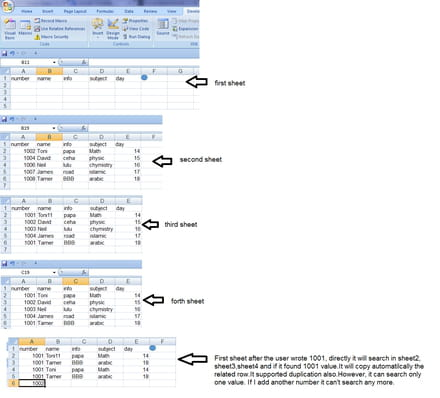# Searching for a specific values on multiple sheets using Macro

Closed
Fajr123 Posts 2 Registration date Saturday February 20, 2016 Status Member Last seen February 20, 2016 - Feb 20, 2016 at 05:14 AM
Fajr123 Posts 2 Registration date Saturday February 20, 2016 Status Member Last seen February 20, 2016 - Feb 20, 2016 at 07:41 AM
Hello,

I have multiple different sheets,
for example I have 4 sheets now.
first sheet,second sheet, third sheet, forth sheet. each sheet has 5 columns that contains information.
I used first sheet for searching As shown in the image.
Open the site please to see my problem.Can anyone fix my code to make it do a search for more than one value?
It needs only one for loop, but I don't know where should i put it..
Help me please as soon as posibble

`Sub SmileyFace1_Click()Dim q, i, j, k, l, m, n, no_sheets As IntegerDim key, cursor, sheetname As StringDim flag As BooleanDim sheet1_row, row_count As Integer    no_sheets = 4    k = 2    sheet1_row = 2        For i = 2 To no_sheets        key = ThisWorkbook.Worksheets("sheet1").Range("A2")        flag = False        sheetname = "Sheet" & i        row_count = WorksheetFunction.CountA(ThisWorkbook.Worksheets(sheetname).Range("A:A"))        For j = 2 To row_count            cursor = ThisWorkbook.Worksheets(sheetname).Range("A" & j)            If key = cursor Then                ThisWorkbook.Worksheets("sheet1").Range("A" & sheet1_row) = ThisWorkbook.Worksheets(sheetname).Range("A" & j)                ThisWorkbook.Worksheets("sheet1").Range("B" & sheet1_row) = ThisWorkbook.Worksheets(sheetname).Range("B" & j)                ThisWorkbook.Worksheets("sheet1").Range("C" & sheet1_row) = ThisWorkbook.Worksheets(sheetname).Range("C" & j)                ThisWorkbook.Worksheets("sheet1").Range("D" & sheet1_row) = ThisWorkbook.Worksheets(sheetname).Range("D" & j)                ThisWorkbook.Worksheets("sheet1").Range("E" & sheet1_row) = ThisWorkbook.Worksheets(sheetname).Range("E" & j)                flag = True                sheet1_row = sheet1_row + 1                            End If        Next j   Next i        If flag = False Then                    ThisWorkbook.Worksheets("sheet1").Range("B" & sheet1_row) = "Not found"                    ThisWorkbook.Worksheets("sheet1").Range("C" & sheet1_row) = "Not found"                    ThisWorkbook.Worksheets("sheet1").Range("D" & sheet1_row) = "Not found"                    ThisWorkbook.Worksheets("sheet1").Range("E" & sheet1_row) = "Not found"        End IfEnd SubSub MatchUnMatch_Click()    Dim i, j, k, l, m, n As Integer    Dim ListA_count, ListB_count, ListC_count, ListD_count, ListE_count As Integer    Dim key, cursor As String    Dim flag As Boolean        ListA_count = WorksheetFunction.CountA(ThisWorkbook.Worksheets("MatchUnmatch").Range("A:A"))    ListB_count = WorksheetFunction.CountA(ThisWorkbook.Worksheets("MatchUnmatch").Range("B:B"))    'ListA_count = ThisWorkbook.Worksheets("MatchUnMatch").Range("A2").End(xlDown).Row    'MsgBox ListA_count & " " & ListB_count    '=======================================================================================================    '    '    ' Matching Logic for List 'A' and List 'B'    '    '    '=======================================================================================================    k = 2    For i = 2 To ListA_count        key = ThisWorkbook.Worksheets("MatchUnMatch").Range("A" & i)                For j = 2 To ListB_count            cursor = ThisWorkbook.Worksheets("MatchUnMatch").Range("B" & j)            'MsgBox "Key=" & Key & " Cursor=" & cursor            If key = cursor Then                ThisWorkbook.Worksheets("MatchUnMatch").Range("C" & k) = key                k = k + 1                Exit For            End If        Next j    Next i        '=======================================================================================================    '    '    ' List 'A' items not in List 'B'    '    '    '=======================================================================================================    ListC_count = WorksheetFunction.CountA(ThisWorkbook.Worksheets("MatchUnmatch").Range("C:C"))        k = 2    For i = 2 To ListA_count        key = ThisWorkbook.Worksheets("MatchUnMatch").Range("A" & i)        flag = False        For j = 2 To ListC_count            cursor = ThisWorkbook.Worksheets("MatchUnMatch").Range("C" & j)            If key = cursor Then                flag = True                Exit For            End If        Next j        If flag = False Then            ThisWorkbook.Worksheets("MatchUnMatch").Range("D" & k) = key            k = k + 1        End If    Next i            '=======================================================================================================    '    '    ' List 'B' items not in List 'A'    '    '    '=======================================================================================================    k = 2    For i = 2 To ListB_count        key = ThisWorkbook.Worksheets("MatchUnMatch").Range("B" & i)        flag = False        For j = 2 To ListC_count            cursor = ThisWorkbook.Worksheets("MatchUnMatch").Range("C" & j)            If key = cursor Then                flag = True                Exit For            End If        Next j        If flag = False Then            ThisWorkbook.Worksheets("MatchUnMatch").Range("E" & k) = key            k = k + 1        End If    Next iEnd Sub`

`Sub SmileyFace1_Click()Dim q, i, j, k, l, m, n, no_sheets As IntegerDim key, cursor, sheetname As StringDim flag As BooleanDim sheet1_count, sheet1_row, row_count As Integersheet1_count = WorksheetFunction.CountA(ThisWorkbook.Worksheets("sheet1").Range("A:A"))    no_sheets = 4    k = 2    sheet1_row = 2For q = 2 To sheet1_count     key = ThisWorkbook.Worksheets("sheet1").Range("A" & q)    For i = 2 To no_sheets        flag = False        sheetname = "Sheet" & i        row_count = WorksheetFunction.CountA(ThisWorkbook.Worksheets(sheetname).Range("A:A"))        For j = 2 To row_count            cursor = ThisWorkbook.Worksheets(sheetname).Range("A" & j)            If key = cursor Then                ThisWorkbook.Worksheets("sheet1").Range("A" & sheet1_row) = ThisWorkbook.Worksheets(sheetname).Range("A" & j)                ThisWorkbook.Worksheets("sheet1").Range("B" & sheet1_row) = ThisWorkbook.Worksheets(sheetname).Range("B" & j)                ThisWorkbook.Worksheets("sheet1").Range("C" & sheet1_row) = ThisWorkbook.Worksheets(sheetname).Range("C" & j)                ThisWorkbook.Worksheets("sheet1").Range("D" & sheet1_row) = ThisWorkbook.Worksheets(sheetname).Range("D" & j)                ThisWorkbook.Worksheets("sheet1").Range("E" & sheet1_row) = ThisWorkbook.Worksheets(sheetname).Range("E" & j)                flag = True                sheet1_row = sheet1_row + 1                q = sheet1_row            End If        Next j   Next i Next q           If flag = False Then                    ThisWorkbook.Worksheets("sheet1").Range("B" & sheet1_row) = "Not found"                    ThisWorkbook.Worksheets("sheet1").Range("C" & sheet1_row) = "Not found"                    ThisWorkbook.Worksheets("sheet1").Range("D" & sheet1_row) = "Not found"                    ThisWorkbook.Worksheets("sheet1").Range("E" & sheet1_row) = "Not found"        End If          End SubSub MatchUnMatch_Click()    Dim i, j, k, l, m, n As Integer    Dim ListA_count, ListB_count, ListC_count, ListD_count, ListE_count As Integer    Dim key, cursor As String    Dim flag As Boolean        ListA_count = WorksheetFunction.CountA(ThisWorkbook.Worksheets("MatchUnmatch").Range("A:A"))    ListB_count = WorksheetFunction.CountA(ThisWorkbook.Worksheets("MatchUnmatch").Range("B:B"))    'ListA_count = ThisWorkbook.Worksheets("MatchUnMatch").Range("A2").End(xlDown).Row    'MsgBox ListA_count & " " & ListB_count    '=======================================================================================================    '    '    ' Matching Logic for List 'A' and List 'B'    '    '    '=======================================================================================================    k = 2    For i = 2 To ListA_count        key = ThisWorkbook.Worksheets("MatchUnMatch").Range("A" & i)                For j = 2 To ListB_count            cursor = ThisWorkbook.Worksheets("MatchUnMatch").Range("B" & j)            'MsgBox "Key=" & Key & " Cursor=" & cursor            If key = cursor Then                ThisWorkbook.Worksheets("MatchUnMatch").Range("C" & k) = key                k = k + 1                Exit For            End If        Next j    Next i        '=======================================================================================================    '    '    ' List 'A' items not in List 'B'    '    '    '=======================================================================================================    ListC_count = WorksheetFunction.CountA(ThisWorkbook.Worksheets("MatchUnmatch").Range("C:C"))        k = 2    For i = 2 To ListA_count        key = ThisWorkbook.Worksheets("MatchUnMatch").Range("A" & i)        flag = False        For j = 2 To ListC_count            cursor = ThisWorkbook.Worksheets("MatchUnMatch").Range("C" & j)            If key = cursor Then                flag = True                Exit For            End If        Next j        If flag = False Then            ThisWorkbook.Worksheets("MatchUnMatch").Range("D" & k) = key            k = k + 1        End If    Next i            '=======================================================================================================    '    '    ' List 'B' items not in List 'A'    '    '    '=======================================================================================================    k = 2    For i = 2 To ListB_count        key = ThisWorkbook.Worksheets("MatchUnMatch").Range("B" & i)        flag = False        For j = 2 To ListC_count            cursor = ThisWorkbook.Worksheets("MatchUnMatch").Range("C" & j)            If key = cursor Then                flag = True                Exit For            End If        Next j        If flag = False Then            ThisWorkbook.Worksheets("MatchUnMatch").Range("E" & k) = key            k = k + 1        End If    Next iEnd Sub`